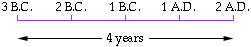Dear math experts; My name is Show_Li. I am a 7th grade student. This is my home work question. The calendar that astronomers use began on Jan. 1, 4713 B.C. on that day, the julian calendar, the lunar calendar, and roman tax system calendar all coincided. This won't happen again until 3267 A.D. (a). How many years ago was the astronomer's calendar started? (b). Find the number of years between times that the three calendars coincided. Thank you for your help. Show_Li Hi Show_Li This is a problem where it is best to start with a small example. Suppose that the calendars coincided on Jan. 1, in the year 3 B.C. an then again in the year 2 A.D. The timeline would look likeWhat you should notice is that 4 years have passed, even though 3 + 2 = 5. The reason is that there was no year zero. The day after December 31 in the year 1 B.C. was January 1, in the year 1 A.D. Thus from Jan. 1, 4713 B.C. to Jan. 1, 3267 A.D. is 4713 + 3267 - 1 = 7979 years. This is the answer to part b. I am presently reading a book which claims that the number of years until the three calendars coincided is 7980 but, according to the numbers that you have here, the number of years is 7979. Penny Go to Math Central